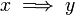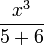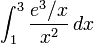# User talk:Emanuele

(Difference between revisions)
 Revision as of 21:09, 14 January 2010 (view source)Emanuele (Talk | contribs)← Older edit Current revision as of 13:43, 15 January 2010 (view source)Emanuele (Talk | contribs) (4 intermediate revisions not shown) Line 1: Line 1: testing math equations testing math equations - $\frac{x}{5}$ + $x \implies y$ - $x^2+3x+5$ + $\frac{x^3}{5+6}$ + + $\int_{1}^{3}\frac{e^3/x}{x^2}\, dx$ + + + --[[User:Emanuele|Emanuele]] 13:43, 15 January 2010 (MST)

## Current revision as of 13:43, 15 January 2010

testing math equations$x \implies y$$\frac{x^3}{5+6}$$\int_{1}^{3}\frac{e^3/x}{x^2}\, dx$

--Emanuele 13:43, 15 January 2010 (MST)